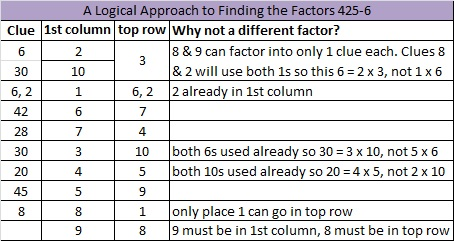# 160 and Level 6

 160 is a composite number. Factor pairs: 160 = 1 x 160, 2 x 80, 4 x 40, 5 x 32, 8 x 20, or 10 x 16 Factors of 160: 1, 2, 4, 5, 8, 10, 16, 20, 32, 40, 80, 160 Prime factorization: 160 = 2 x 2 x 2 x 2 x 2 x 5, which can also be written 160 = (2^5) x 5160 = 2 + 3 + 5 + 7 + 11 + 13 + 17 + 19 + 23 + 29 + 31 (the sum of the first eleven prime numbers).Excel file of puzzles and previous week’s factor solutions: 10 Factors 2014-06-23